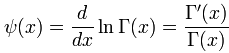## Digamma Function Calculator

 X
 Result: Gamma (x) Γ(X)=

Digamma Function Calculator to calculate the logarithmic derivative of the gamma function.

The symbol of Digamma function is Ψ.

### The digamma function formulawhere

Γ is the gamma function

Γ' is the derivative of the gamma function.

For example, when X = 2, then Gamma (x) Γ(X) = 0.4228.

Thinkcalculator.com provides you helpful and handy calculator resources.